# Polynomial chaos basis¶

The current section is focused on a specific kind of functional chaos representation that has been implemented, namely polynomial chaos expansions.
Mathematical framework
Throughout this section, the model response is assumed to be a scalar random variable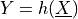. However, the following derivations hold in case of a vector-valued response.
Let us suppose that:
•has a finite variance, i.e.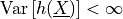;

•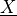has independent components.

Then it is shown that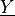may be expanded onto the PC basis as follows:

(1)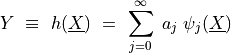where the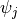’s are multivariate polynomials that are orthonormal with respect to the joint PDF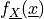, that is: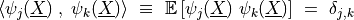where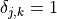if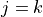and 0 otherwise, and the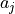’s are deterministic coefficients that fully characterize the response.

Building of the PC basis – independent random variables
We first consider the case of independent input random variables. In practice, the components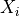of random vectorare rescaled using a specific mapping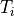, usually referred to as an isoprobabilistic transformation (see ). The set of scaled random variables reads:

(2)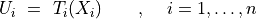Common choices for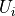are standard distributions such as a standard normal distribution or a uniform distribution over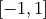. For simplicity, it is assumed from now on that the components of the original input random vectorhave been already scaled, i.e.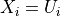.

Let us first notice that due to the independence of the input random variables, the input joint PDF may be cast as:

(3)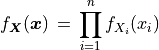where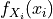is the marginal PDF of. Let us consider a family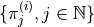of orthonormal polynomials with respect to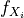, :

(4)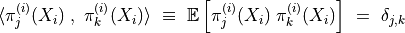The reader is referred to  for details on the selection of suitable families of orthogonal polynomials. It is assumed that the degree of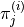is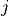for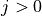and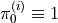(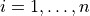). Upon tensorizing the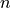resulting families of univariate polynomials, one gets a set of orthonormal multivariate polynomials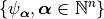defined by:

(5)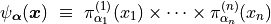where the multi-index notation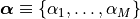has been introduced.

Building of the PC basis – dependent random variables

In case of dependent variables, it is possible to build up an orthonormal basis as follows:

(6)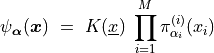where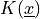is a function of the copula of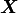. Note that such a basis is no longer polynomial. When dealing with independent random variables, one gets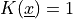and each basis element may be recast as in (5). Determiningis usually computationally expensive though, hence an alternative strategy for specific types of input random vectors.

Ifhas an elliptical copula instead of an independent one, it may be recast as a random vector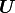with independent components using a suitable mapping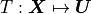such as the Nataf transformation. The so-called Rosenblatt transformation may also be applied in case of a Gaussian copula . Thus the model response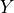may be regarded as a function ofand expanded onto a polynomial chaos expansion with basis elements cast as in (5).
Link with classical deterministic polynomial approximation

In a deterministic setting (i.e. when the input parameters are considered to be deterministic), it is of common practice to substitute the model function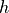by a polynomial approximation over its whole domain of definition as shown in . Actually this approach is strictly equivalent to:

1. Regarding the input parameters as random uniform random variables

2. Expanding any quantity of interest provided by the model onto a PC expansion made of Legendre polynomials